Main Page > SSD projects > Assembly Technology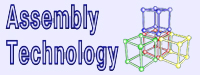### Assembly Technology Project

 Kraeva M.A., Malyshkin V.E. Assembly Technology for Parallel Realization of Numerical Models on MIMD-Multicomputers. In the special issue of the International Journal on Future Generation Computer Systems,devoted to Parallel Computing Technologies. Vol. 17 (2001), No. 6, pp. 755-765

### 1. Introduction

Parallel implementation of realistic numerical models, using direct modeling of a physical phenomenon on the basis of description of the phenomenon behaviour in the local area, usually requires high performance computations. However these models are also remarkable for irregularity and even dynamically changing irregularity of the data structure (adaptive mesh, variable time step, particles, etc.). For example, in the PIC method the test particles are in the bottom of such an irregularity. Additionally, parallel programs, implemented realistic numerical models, should possess by new dynamic properties like dynamic load balancing. Hence, these models are very difficult for effective parallelization and high performance implementation with conventional programming systems.
The Assembly Technology (AT) [1-3] has been especially created in order to support the development of fragmented parallel programs for multicomputers. Fragmentation and automatic dynamic load balancing are the key features of programming and program execution under the AT. In order to provide high performance of target programs the AT is implemented in different ways for different application areas. In this paper, the application of the AT to implementation of big size numerical models is demonstrated on the example of parallel implementation of the PIC method [4,5].

### 2. The PIC method and the problems of its parallel implementation

The particle simulation is a powerful tool for modeling of the behaviour of complex non-linear phenomena in plasmas and fluids. In the PIC method, trajectories of a huge number of test particles are calculated as these particles are moved under the influence of the electromagnetic fields computed self-consistently on a discrete mesh. These trajectories represent a desirable solution of the system of differential equations describing a physical phenomenon under study.
A real physical space is represented by a model of simulation domain called the space of modeling (SM). The electric E and magnetic B fields are defined as vectors and discretised upon rectangular mesh (or several shifted meshes, as shown in Figs.1 and 2). Thus, as distinct from other numerical methods on the rectangular mesh, in the PIC method there are two different data structures – particles and meshes. None of the particles affects another particle. At any moment of modeling a particle belongs to a certain cell of each mesh.
Each charged particle is characterized by its mass, co-ordinates and velocities. Instead of solution of equations in the 6D space of co-ordinates and velocities, the dynamics of the system is determined by integrating the equations of motion of every particle in the series of discrete time steps. At each time step tk+1:=tk+Δt the following is done:

1. for each particle, the Lorentz force is calculated from the values of electromagnetic fields at the nearest mesh points (gathering phase);
2. for each particle the new co-ordinates and velocity of a particle are calculated; a particle can move from one cell to another (moving phase);
3. for each particle the charge carried by a particle to the new cell vertices is calculated to obtain the current charge and density, which are also discretised upon the rectangular mesh (scattering phase);
4. Maxwell's equations are solved to update the electromagnetic field (mesh phase).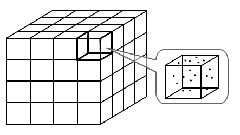Fig. 1. A cell of the SM with the electric E and magnetic B fields, discretised upon shifted meshes Fig. 2. The whole space of modeling (SM) assembled out of cells

The sizes of a time step and of a cell are chosen in such a manner that a particle cannot fly farther than into the adjacent cell at one time step of modeling. The number of time steps depends on a physical experiment. The PIC algorithm has the great possibility for the parallelisation, because all the particles are moved independently. The volume of computations at the first three phases of each time step is proportional to the number of particles. About 90% of multicomputer resources are spent for the particle processing. Thus, in order to implement the PIC code on multicomputer with high performance, the equal number of particles should be assigned for processing to each processor element (PE). However, on the MIMD distributed memory multicomputers, the performance characteristics of the PIC code crucially depends on how the mesh and particles are distributed among the PEs. In order to decrease the communication overheads at the first and the third phases of a time step, it is required that a PE contains as the cells (values of the electromagnetic fields at the mesh points) as the particles located inside them. Unfortunately, in the course of modeling, some particles might fly from one cell to another. To satisfy the previous requirement, two basic decompositions can be used [4,5]. In the so-called Lagrangian decomposition, the equal number of particles are assigned to each PE with no regard for their position in the SM. In this case, the values of the electromagnetic fields, the current charge and density at all the mesh points should be copied in every PE. Otherwise, the communication overheads at the first and the third phases will decrease the effectiveness of parallelisation. Disadvantages of the Lagrangian decomposition are the following:

• strict memory requirements;
• communication overheads at the second phase (to update the current charge and the current density in each PE).

In the Eulerian decomposition, each PE contains a fixed rectangular sub-domain, including electromagnetic fields at the corresponding mesh points and particles in the corresponding cells. If a particle leaves its sub-domain and flies to another sub-domain in the course of modeling, then this particle should be transferred to the PE containing this latter sub-domain. Thus, even with an equal initial workload of the PEs, in several steps of modeling, some PEs might contain more particles than the others. This results in the load imbalance. The character of the particles motion does not fully depend on equations, but also on the initial particles distribution and the initial value of the electromagnetic field.
Let us consider some examples of particles distribution, which correspond to the different real physical experiments.

• Uniform distribution of the particles in the entire space of modeling.
• The case of a plate. The space of modeling has n1 x n2 x n3 size. The particles are uniformly distributed in k x n2 x n3 size space (k<<n1).
• Flow. The set of particles is divided into two subsets: the particles with zero initial velocity and the active particles with the initially nonzero velocity. Active particles are organized as a flow crossing the space along a certain direction.
• Explosion. There are two subsets of particles. The background particles with zero initial velocities are uniformly distributed in the entire space of modeling. All the active particles form a symmetric cloud (r<<h, where r is the radius of the cloud, h is the mesh step). The velocities of active particles are directed along the radius of the cloud.

The main problem of programming is that the data distribution among the PEs depends not only on the volume of data, but also on the data properties (particles velocities, configuration of electromagnetic field, etc.). With the same volume of data but different particles distributions inside the space the data processing is organized in different ways.
As the particles distribution is not stable in the course of modeling, the program control and data distribution among the PEs should be dynamically changing. It is clear that the parallel implementation of the PIC method on a distributed memory multicomputer strongly demands the dynamic load balancing.

### 3. Substantial features of AT

Numerical methods, generally, and the PIC method, in particular, are very suitable for the application of AT. The fragmented representation of an algorithm affects different stages of an application program development. Here we would like in brief to list some peculiarities of the AT.

3.1 Algorithm and program fragmentation
In accordance with the AT, the description of an application problem should be divided into a system of reasonably small atomic fragments, representing the implementation entities of a problem description. Fragments might be represented in programming languages by variables, procedures, subroutines, macros, nets, notions, functions, etc. depending on the method of the AT implementation. The program, which realizes an atomic fragment (P_fragment), contains both data and code. In other words, a program, realizing an application problem, is assembled out of such small P_fragments of computations which are connected through variables for data transfer.
The fragmented structure of an application parallel program is kept in the executable code and provides the possibility for organization of flexible and high performance execution of the assembled program. The general idea is the following. An assembled program is represented in a programming language, and is composed as a system of executable P_fragments. This program is executed inside every PE, looping over all the P_fragments, loaded to the PE. If these fragments are small enough, then initially for each PE of a multicomputer the equal workload is assembled out of these P_fragments. This is of course a general idea only. Actually, in order to attain high performance of a target program its assembling is realized in different ways.
The workload of PEs can be changed in the course of computation, and if at least one PE becomes overloaded, then a part of P_fragments (with the processing data), which were assigned for execution into the overloaded PE, should fly to the underloaded PEs equalizing the workload of multicomputer PEs. Dynamic load balancing of a multicomputer is based on such a fragmentation [5,6]. The algorithms of a target program assembling out of atomic fragments are not discussed here in detail.

3.2 Assembling vs. Partitioning
Our basic key word is assembly. Contrary to partitioning, the AT supports explicit assembling of a whole program out of ready-made fragments of computations, rather then dividing a problem, defined as a whole, into the suitable fragments to be executed on the different PEs of a multicomputer. These fragments are the elementary blocks, the "bricks", to construct a whole program (a whole problem description), there is no a difference here). An algorithm of a problem assembling is kept and used later for dynamic program/problem parallelization. Assembling defines the "seams" of a whole computation, the way of the fragments connection. Therefore, these seams are the most suitable way to cut the entire computation for parallel execution. For this reason program parallelization can be always done if the appropriate size of atomic fragments is chosen.

3.3 Explicitly two-level programming
Under the AT, first, suitable atomic fragments of computation are designed, programmed and debugged separately. Then the whole computation (problem solution) is assembled out of these fragments.

3.4 Separation of the fine grain and the coarse grain computations.
The fine and the coarse grain computations are executed on the different level of hardware and software. Under AT the fine grain computations are encapsulated inside a module (P_fragment) that realizes the computations bound up with atomic fragment. Such a module can be implemented very effectively on a processor element. The whole parallel program is assembled out of these ready-made modules. The system of modules of a program defines a system of interacting processes (coarse grain computations). Encapsulation of the fine grain computations, their complexity, inside an atomic fragment provides the possibility of formalization of a parallel program construction and the use of explicitly two-level representation of an algorithm: the programming level inside an atomic fragment and the scheme level of an application program assembling.

3.5 Separation of semantics and scheme of computation
The fine grain computations define a sufficient part of semantics (functions) of computations. They are realized within an atomic fragment. Therefore, on the coarse grain level, only a scheme (non-interpreted or semi-interpreted) of computations is assembled. It means that formal methods of automatic synthesis of parallel programs  can be successfully used and the standard schemes of parallel computations can be accumulated in libraries.

### 4. Parallelization of numerical methods with AT

Let us consider the assembly approach to the numerical methods parallelisation on the example of the PIC method parallel implementation.
The line and the 2D grid structure of interprocessor communications of a multicomputer are sufficient for the effective parallel implementation of numerical methods on the rectangular meshes. In  the algorithm of the 2D grid mapping into the hypercube keeping the neibourhood of PEs is given.
A cell is natural atomic fragment of computation for a numerical method implementation. It contains both data (particles inside the cells of a fragment and values of electromagnetic fields, current density at their mesh points) and the procedures, which operate with these data. For the PIC method, when a particle moves from one cell to another, it should be removed from the former cell and added to the latter cell. Thus, we can say that with the AT the Eulerian decomposition is realized.

4.1 PIC parallelization on the line of PEs
Let us consider first in what way the PIC is parallelized for the multicomputers with the line structure of interprocessor communications. The three-dimensional simulation domain is initially partitioned into N blocks (where N is the number of PEs). Each block_i consists of several adjacent layers of cells and contains approximately the same number of particles (Figs. 3, 4). When the load imbalance is crucial, some layers of the block located into an overloaded PE are transferred to another less loaded PE. In the course of modeling, the adjacent blocks are located in the linked PEs. Therefore, the adjacent layers are located in the same or in the linked PEs. It is important for the second phase, at which some particles can fly from one cell into another and for the fourth phase, when for recalculation of values of electromagnetic fields in a certain cell, values in the adjacent cells are also used.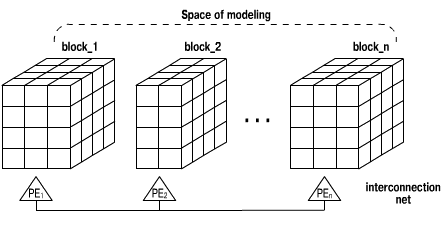Fig. 3. Decomposition of SM for implementation of the PIC on the line of PEs

4.2 PIC parallelization on the 2D grid of PEs
Let us consider now the PIC method parallelization for the 2D grid of PEs. Let the number of PEs be equal to lxm. Then SM is divided into l blocks orthogonal to a certain axis. Each block consists of several adjacent layers and contains about NP/l particles (in the same way as it was done for the line of PEs). The block_i is assigned for processing to the i-th row of the 2-D grid (Fig. 4). Blocks are formed in order to provide an equal total workload of every PE's row of the processor grid. Then every block_i is divided into m sub-blocks block_i_j, which are distributed for processing among m PEs of the row. These sub-blocks are composed in such a way in order to provide an equal workload of every PE of the row. If overload of at least one PE occurs in the course of modeling, this PE is able to recognize it at the moment when the number of particles substantially exceeds NP/(lxm). Then this PE initiates the re-balancing procedure.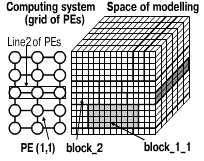Fig. 4. Decomposition of SM for implementation of PIC on the 2D grid of PEs

If the number of layers k x N (or k x l in the case of the grid of PEs), it is difficult or even impossible to divide the SM into blocks with the equal number of particles. Also, if particles are concentrated inside a single cell, it is definitely impossible to divide SM into the equal sub-domains. In order to attain the better load balance, the following modified domain decomposition is used. A layer containing more than the average number of particles is copied at least into 2 or more neighbouring PEs (Fig. 5) √ these are virtual layers. A set of particles located inside such a layer are distributed among all these PEs. In the course of the load balancing, particles inside the virtual layers are the first to be redistributed among PEs, and only if necessary, the layers are also redistributed. For the computations inside a PE, there is no difference between virtual and non-virtual layers. Any layer could become virtual in the course of modeling, a virtual layer can stop to be virtual.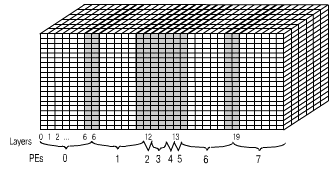Fig. 5. Virtual layers for implementation of PIC on the 2D grid of PEs

We can see, that in both cases there is no a necessity to provide flying of a cell. A cell is very small fragment, therefore a large resources should be spent to provide its flying. Thus, there is a necessity to use bigger indivisible fragments on the step of execution (not on the step of a problem/program assembling!). In the case of the line of PEs a layer of the SM should be chosen as indivisible fragment of concrete implementation of PIC. Such a fragment is called a minimal fragment. For PIC implementation on the grid of PEs a column is taken as minimal indivisible fragment. Procedure realizing minimal fragment is composed statically out of P_fragments, before the whole program assembling. This essentially improves the performance of an executable code.
In such a way, a cell is used as atomic fragment at the step of numerical algorithm description. At the step of execution of a numerical algorithm, different minimal fragments assembled out of atomic fragments are chosen depending on architecture of a multicomputer.

4.3 Implementation of the PIC method on multicomputers
Using AT PIC method has been implemented on different multicomputer systems (MBC-100, multitransputer system, Hypercube Intel iPSC/860, Parsytec PowerXplorer, Cray J90, IBM SP2). In order to provide a good portabilitym the language C was chosen for the parallel PIC code implementation. Since different message passing systems are used for different multicomputers, the special communication functions were designed. In the compilation time the actual functions of a target multicomputer are substituted instead of these communication functions. In particular, the MPI functions might be substituted. For the dynamic load balancing of PIC several algorithms were developed [7,8]. In the cases of the grid communication structure (Fig. 6) and the virtual fragments (particles might fly not only to the neighbouring PEs) special tracing functions are used.Fig. 6. Direction of data transfer for implementation of the PIC on the 2D grid

According to the AT the array of particles is divided into N parts, where N is the number of minimal fragments (layers of SM in the case of the line of PEs, and columns in the case of the 2D grid). When elements of the mesh variables (electromagnetic fields, current charge and density) of different minimal fragments hit upon the same point of SM, they are unified (Fig. 7). The elements of the mesh variables of one minimal fragment are not added to the data structure for this fragment, but are stored in the 3D arrays with elements of the mesh variables of other minimal fragments in the PE. This appears possible due to the rectangular shape of blocks block_i (block_i_j in the case of the 2D grid). Such a decision allows us to decrease the memory requirements and to speed up computations during the forth phase of the PIC algorithm.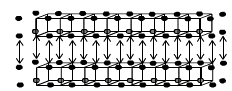Fig. 7. Unification of the Hx mesh variables for two minimal fragments (layers)

In the case of dynamic load balancing, when some minimal fragments are transferred from one PE to another, the size of the 3D arrays of elements of the mesh variables is dynamically changed. This demands special implementation of such dynamic arrays.

To attain a high performance of parallel PIC implementation, a number of centralized and decentralized load balancing algorithms were especially developed.

A layer of cells is chosen as minimal fragment for the PIC implementation on the line of PEs. Each minimal fragment has its own weight. The weight of a minimal fragment is equal to the number of particles in this fragment. The sum of weights of all the minimal fragments in some PE determines the workload of PE. The 3D simulation domain is initially partitioned with a certain algorithm into N blocks (where N is the number of PEs). Each block consists of several adjacent minimal fragments and contains approximately the average number of particles. For the initial load balancing two heuristic centralized algorithms were designed [7,8]. These algorithms employ the information about the weights of all the minimal fragments. Each PE has this information and constructs the workload card by the same algorithm. The workload card contains the list of minimal fragments that should be loaded to the PEs. If the number of minimal fragments is much greater than the number of PEs, it is usually possible to distribute the minimal fragments among the PEs in such a way that every block would contain approximately the same number of particles.
If considerable portion of particles is concentrated inside a single cell, it is impossible to divide the SM into the blocks with quite an equal workload. To solve this problem, a notion of virtual layer is introduced. The centralized algorithm was modified for the case of virtual fragments.
If overloading of at least one PE occurs in the course of modeling, this PE is able to recognize it at the moment when the number of particles substantially exceeds NP/N. Then this PE initiates the re-balancing procedure.

If a load imbalance occurs, the procedure BALANCE is called. In this procedure, the decision about the direction of data transfer and the volume of data to be transferred is taken. For any load balancing algorithm there exists its own realizing procedure BALANCE. The procedure TRANSFER is used for the data transfer. There are two implementations of this procedure: for the line of PEs and for the grid of PEs. The procedure TRANSFER is the same for any load-balancing algorithm on the line of PEs. Parameters of the procedure are the number of particles to be exchanged and the direction of data transfer.
Let us consider algorithms of the dynamic load balancing of the PIC. All the PEs are numerated. In the case of the line of PE, each PE has number i, where 0<= i < number_of_PEs. In the case of the (l x m) grid of PEs, the number of PE is the pair (i,j) , where 0 <=i < l, 0 <=j < m. In the same way, layers and columns of the SM are numerated.

5.3 Centralized dynamic load balancing algorithm
For the dynamic load balancing the initial load balancing algorithm could be used. One of PEs collects the information about weights of the minimal fragments and broadcasts this information to all the other PEs. All the PEs build the new load card. After that neighbouring PEs exchange minimal fragments according to the information in the new workload card.
Imbalance Threshold. If centralized algorithms are used for the dynamic load balancing, the PEs exchange the information about load balancing, therefore every PE has information about the number of particles in all the PEs. In any PE, the difference mnp-NP/N (where mnp is the maximum number of particles in PE, NP is the total number of particles, N is the number of PEs) is calculated. If the difference is more than the threshold Th, the procedure BALANCE is called. The threshold can be a constant, chosen in advance, or an adaptive number. In the latter case, initially Th=0. In the course of modeling, the time t_part, which is required to implement steps 1)-3) of the PIC algorithm for one particle, is calculated. After every BALANCE call the time of balancing t_bal is calculated. Th is assigned to be equal to t_bal/t_part (how many particles could be processed for the same time as one balancing requires). After each subsequent step of the PIC algorithm, Th is decreased by mnp-NP/N. When the value of Th is negative, BALANCE is called.
If the threshold is always equal to zero, the procedure BALANCE is called after each time step of modeling.

5.4 Decentralized dynamic load balancing algorithm for the constant number of particles
The use of centralized algorithms is good enough for multicomputers containing a few PEs. But if the number of PEs is big enough, the communication overheads could neutralize the advantages of the dynamic load balancing. In this case it is more preferable to use decentralized algorithms. Such algorithms use information about the load balance in the local domain of PEs only.
If the number of model particles is not changed in the course of modeling, a simple decentralized algorithm could be suggested. Each PE has the information on how many particles were moved to/from its neighbouring PEs. To equalize the load balance it is sufficient just to receive/send the same number of particles from/to the neighbouring PEs. It should be noted that this algorithm works in the case of virtual fragments only.

5.5 Specialized decentralized algorithm
To equalize the load balance for the PIC implementation, the following specialized algorithm was designed. In the course of modeling in every PE the main direction of particles motion is calculated from the values of particles velocities. In the load balancing, particles are delivered to the direction opposite to the main direction. As in the previous algorithm it is assumed that the number of particles is not changed in the course of modeling. The number of particles to be transferred from overloaded PEs to their neighbours in the direction opposite to the main direction is calculated from the average number of particles and the number of particles in the PE. Some particles are transferred in advance, in order to reduce the number of calls of the dynamic load balancing procedure.

The basic diffusive load-balancing algorithm was implemented and tested for the PIC parallel implementation [7-9]. The size of the local domain is equal to two. Any diffusive algorithm is characterized by the number of steps. Actually the number of steps defines how far the data from one PE could be transferred in the course of load balancing. For every step the procedure TRANSFER is called. The more the number of steps of diffusive algorithm the better load balance could be attained, but also the more time is required for load balancing. The tests have shown that the total program time does not decrease with the growth of the number of steps.

5.7 Algorithms comparison
In the following Tables the results of different dynamic load balancing algorithms testing for the problem of plasma cloud explosion modeling are shown. The size of the problem is the following:
∙ The size of the space of modeling - 24x24x36
∙ The number of background particles - 24*24*36*27=559872
∙ The number of cloud particles - 240128
∙ The total number of particles - 800

 2 PE 3 PE 4 PE 5 PE 6 PE 7PE 8 PE 20 PE The max number of particles in PE 400000 353256 223190 207663 213364 153948 152389 79930 Duration of particles processing 110190 97462 62012 57375 59072 42821 46980 22267 Total time 121768 107878 68801 63481 65332 47470 46716 24687 Tab.1 Dynamic load balancing is absent

 2 PE 3 PE 4 PE 5 PE 7 PE 8 PE The max number of particles in PE 400000 266787 200102 160091 114388 100091 Duration of particles processing 110625 73862 55722 44498 32189 27579 Total time 122294 81900 61902 49453 35826 30744 Tab.2 Centralized load balancing algorithm

 2 PE 3 PE 4 PE 5 PE 6 PE 7PE 8 PE 20 PE The max number of particles in PE 400000 266977 200001 160484 133679 153948 100727 42092 Duration of particles processing 110459 75116 56246 45036 59072 37270 28260 11887 Total time 122048 83191 62606 50083 65332 47470 31508 13353 Tab.3 Diffusive load balancing algorithm (the number of steps is equal to 2)

 2 PE 3 PE 4 PE 5 PE 6 PE 7 PE 8 PE 20 PE The max number of particles in PE 400000 328325 200000 161630 133473 115489 100238 41388 Duration of particles processing 110329 90333 56203 45926 36821 32669 28504 11647 Total time 121952 100022 62337 50946 40980 36419 31801 13093 Tab. 4 Specialized decentralized algorithm

If the number of PEs is greater than 2, the load balancing is required. The results of the tests confirm the fact, that for the small number of PEs (less than 10), the utilization of a centralized algorithm is more preferable, though decentralized algorithms also give satisfactory results.

### 6. Automatic generation of parallel code

The PIC method is applied to simulation of many natural phenomena. In order to facilitate the parallel PIC implementation, a special system of parallel program automatic generation was designed. This system consists of the VISual system of parallel program Assembling (VisA) and a parallel code generator in C language.
The process of the generation of a parallel program for the PIC and for the other numerical algorithms on the rectangular meshes consists of three steps.
At the first step, a user defines the atomic fragment of computation √ a cell of the SM. This cell contains elements of the mesh variables at several mesh points, an array of particles (for PIC method) and procedures in C language, which describe all the computations inside the cell √ {procedure1,┘,procedurek} (Fig. 8).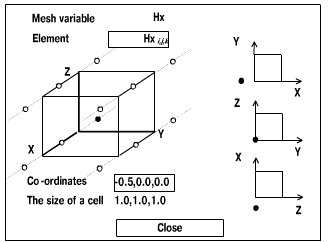Fig. 8. Review window for Hx mesh variable of an atomic fragment of computation

On the next step the user chooses the target multicomputer and the topology of the communication net, for which the parallel code should be generated.
After that the generator constructs a minimal fragment (a layer or a column) from the atomic fragments according to the chosen communication topology. The particle arrays of atomic fragments merge to a single particle array for the minimal fragment. The elements of the mesh variables, which hit upon the same point of SM, are unified. In such a way, for every mesh variable, only one 3D array of its elements is formed.
At the third step, the decision on the PEs workload is made. The generator creates a parallel program for a target multicomputer. This program includes data initialization and a time loop. At each iteration of the time loop, k loops (where k is the number of procedures in the description of an atomic fragment) over all the minimal fragments of PE run. After each k-th loop, those elements of the mesh variables, which are copied in several PEs, are updated (if necessary).
All the particles are stored in m arrays (where m is the number of minimal fragments in a certain PE). However, similarly the case of the minimal fragment assembling, the elements of a mesh variable in all the minimal fragments of a PE form one 3D array.
The user develops procedures (computations inside a cell) in C language, using also several additional statements for defining the computations over the mesh variables.

### 7. Conclusion

The described algorithms of the numerical models assembling provide high performance of the assembled program execution, its high flexibility in reconstruction of the code and dynamic tunability to available resources of a multicomputer.
High performance of the program execution provides the modeling of big size problems such as the study of a cloud plasma explosion in the magnetized background, modeling of interaction of a laser impulse with plasma, etc.
We apply the assembly technology to implementation of different numerical methods and hope to create a general tool to support implementation of mathematical approximating models.

Publications:
1. V.A.Valkovskii, V.E.Malyshkin. Synthesis of Parallel Programs and Systems on the Basis of Computational Models.// Nauka, Novosibirsk, 1988. (In Russian)
2. V.Malyshkin. Functionality in ASSY System and Language of Functional Programming.// Proc. "The First Aizu International Symposium on Parallel Algorithms/Architecture Synthesis", 1995, Aizu-Wakamatsu, Fukushima, Japan. IEEE Comp. Soc. Press, Los Alamitos, California. P. 92-97.
3. Kraeva M.A., Malyshkin V.E. Assembly Technology for Parallel Realization of Numerical Models on MIMD-Multicomputers. In the special issue of the International Journal on Future Generation Computer Systems, devoted to Parallel Computing Technologies. Vol. 17 (2001), No. 6, pp. 755-765.
4. M.Kraeva, V.Malyshkin. Implementation of PIC Method on MIMD Multicomputers with Assembly Technology.// Proc. of HPCN Europe 1997 (High Performance Computing and Networking) Int. Conference, LNCS, Vol.1255, Springer Verlag, 1997. pp. 541-549.
5. M.A.Kraeva, V.E.Malyshkin. Algorithms of Parallel Realization of PIC Method with Assembly Technology. In Proceedings of 7th HPCN Europe 1999, Springer Verlag, LNCS, Vol. 1593, pp.329-338.
6. M.Kraeva, V.Malyshkin. Dynamic load balancing algorithms for implementation of PIC method on MIMD multicomputers.// Programmirovanie, 1999, N 1, p.47-53 (in Russian)

Supervisor: Malyshkin V. E. ( )
Ph. D. Student: Nechaev S. P. ( )